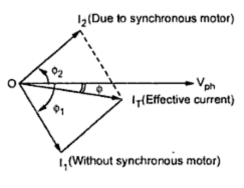### Synchronous Condensers

When synchronous motor is over excited it takes leading p.f. current. If synchronous motor is on no load, where load angle δ is very small and it is over excited (Eb > V) then power factor angle increases almost upto 90o. And motor runs with almost zero leading power factor condition. This is shown in the phasor diagram Fig. 1.Fig. 1 Synchronous condenser
This characteristics is similar to a normal capacitor which takes leading power factor current. Hence over excited synchronous motor operating on no load condition is called as synchronous condenser or synchronous capacitor. This is the property due to which synchronous motor is used as a phase advancer or as power improvement device.
1.1 Disadvantage of Low Power Factor
In various industries, many machines are of induction motor type. The lighting and heating loads are supplied through transformers. The induction motors and transformers draw lagging current from the supply. Hence the overall power factor is very low and lagging in nature.
The power is given by,
P = VI cosΦ                                         .............. single phase
...                     I = P/(VcosΦ)
The supply voltage is constant and hence for supplying a fixed power P, the current is inversely proportional to the p.f. cosΦ. Let P = KW is to be supplied with a voltage of 230 V then,
Case i) cosΦ = 0.8,
I = (5 x103)/(230 x 0.8) = 27.17 A
Case ii) cos = 0.6,

I = (5 x103)/(230 x 0.6) = 36.23 A
Thus as p.f. decreases, becomes low, the current drawn from the supply increases to supply same power to the load. But if p.f. maintained high, the current drawn from supply is less.
The high current due to low p.f. has following disadvantages :
1. For higher current, conductor size required is more which increases the cost.
2. The p.f. is given by
cosΦ = Active power/ Apparent = (P in KW)/ (S i.e. KVA rating)
Thus for fixed active power P, low p.f. demands large KVA rating alternators and transformers. This increases the cost.
3. Large current means more copper losses and poor efficiency.
4. Large current causes large voltage drops in transmission lines, alternators and other equipments. This results into poor regulation. To compensate such drop extra equipments is necessary which further increases the cost.
Note : Hence power factor improvement is must practice. Hence the supply authorities encourage consumers to improve the p.f.
1.1 Use of Synchronous Condenser in Power Factor Improvement
The low power factor increases the cost of generation, distribution and transmission of the electrical energy. Hence such low power factor needs to be corrected. Such power factor correction is possible by connecting synchronous motor across the supply and operating it on no load with over excitation.
Now let Vph is the voltage applied and I1ph is the current lagging Vph by angle Φ1. This power factor Φ1 is very low, lagging.
The synchronous motor acting as a synchronous condenser is now connected across the same supply. This draws a leading current of I2ph.
The total current drawn from the supply is now phasor of Iph and I2ph. This total current IT now lags Vph by smaller angle Φ due to which effective power factor gets improved. This is shown in the Fig. 2.Fig. 2  Power factor correction by synchronous condenser

This is how the synchronous motor as a synchronous condenser is used to improve power factor of the combined load.